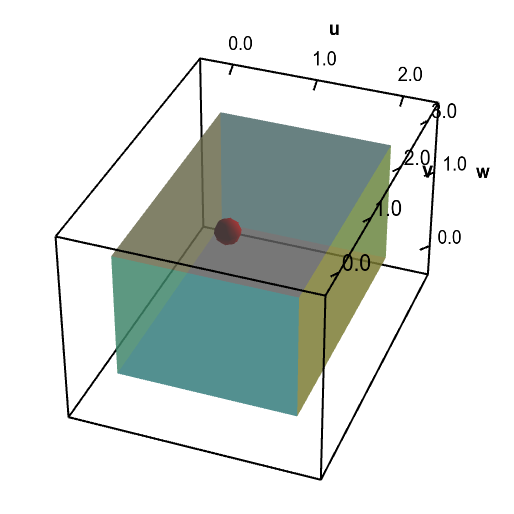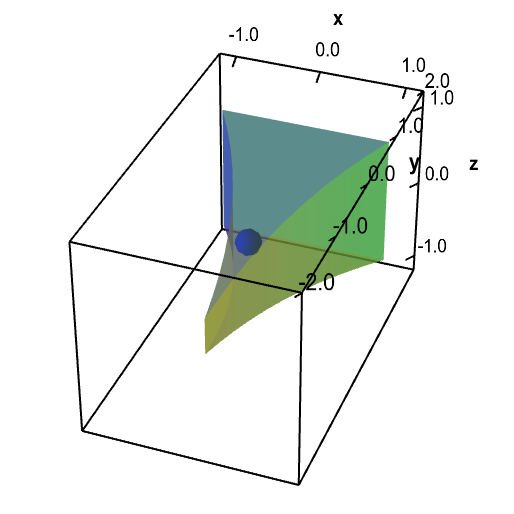# Math Insight

### Applet: A change of variables for an electrode tip domainA change of variables $\cvarf$ maps a rectangular solid $\dlv^*$ (first panel) onto the electrode tip $\dlv$ (second panel). You can explore the mapping by moving with the mouse the red point in $\dlv^*$, which represents the point $(\cvarfv,\cvarsv,\cvartv)$, or the point blue point in $\dlv$, which represents the point $(x,y,z)$. When you move one point, the other point moves to reflect the mapping so that $(x,y,z)= \cvarf(\cvarfv,\cvarsv,\cvartv)$.